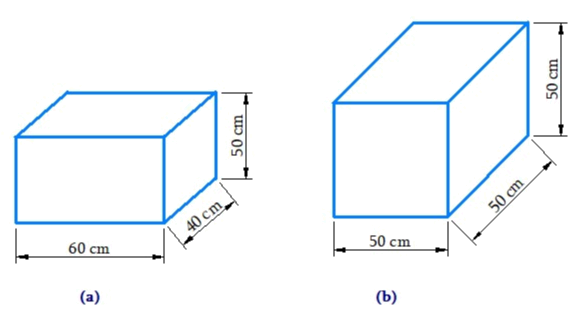# Ex.11.3 Q1 Mensuration Solution - NCERT Maths Class 8

Go back to  'Ex.11.3'

## Question

There are two cuboidal boxes as shown in the adjoining figure. Which box requires the lesser amount of material to make?Video Solution
Mensuration
Ex 11.3 | Question 1

## Text Solution

What is Known?

Dimensions of the cuboidal boxes.

What is unknown?

Surface area of the given figure

Reasoning:

The amount of material requires to make boxes will be equal to their respective surface area.

Steps:

Total surface area of the cuboid lateral surface $$=$$ area of curved surface $$+ 2\; \times$$ area of base

Total surface area of the cub $$= 6{(l)^2}$$

Total surface area of cuboid in figure

\begin{align} &= \! (hl \! + \! hb \! + \! hl \! + \! hb) \! + \! 2(lb)\\&= \! 2(hl \! + \! lb \! + \! hb)\\&= \! 2(60 \! \times \! 50 \! \times \! + \! 40 \! \times \! 50 \! + \! 60 \! \times \! 40)\,\rm{cm^2}\\&= \! 2(3000 \! + \! 2000 \! + \! 2400)\,\rm{cm^2}\\&= \! 14800\,\rm{m^2} \end{align}

Total surface area of cube in figure (b)

\begin{align} &= 6 \times {(l)^2}\\& = 6 \times {(50)^2}\\& = 6 \times 2500\\&= 15000\,\rm{cm^2}\end{align}

Thus, the cuboid box (a) requires lesser amount of material.

Learn from the best math teachers and top your exams

• Live one on one classroom and doubt clearing
• Practice worksheets in and after class for conceptual clarity
• Personalized curriculum to keep up with school